# High School Math : How to find circumference

## Example Questions

← Previous 1

### Example Question #1 : How To Find Circumference

A circle has radius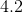. What is the circumference, rounded to the nearest tenth?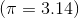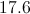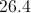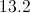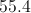Explanation:

Circumference is given by the equation. We can use this equation with the given radius, 4.2, to solve for the circumference.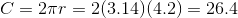### Example Question #2 : How To Find Circumference

What is the circumference of a circle with a radius of 12?

What is the circumference of a circle with a radius of 12?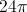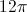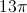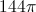Explanation:

To find the circumference of a circle given the radius we must first know the equation for the circumference of a circle which is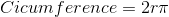We then plug in the number for the radius into the equation yielding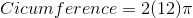We multiply to find the value for the circumference is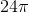.

The answer is.

### Example Question #3 : How To Find Circumference

A circle with an area of 13π in2 is centered at point C. What is the circumference of this circle?

√13π

26π

2√13π

13π

√26π

2√13π

Explanation:

The formula for the area of a circle is πr2.

We are given the area, and by substitution we know that 13π πr2.

We divide out the π and are left with 13 = r2.

We take the square root of r to find that r = √13.

We find the circumference of the circle with the formula = 2πr.

We then plug in our values to find = 2√13π.

### Example Question #4 : How To Find Circumference

A 6 by 8 rectangle is inscribed in a circle. What is the circumference of the circle?

8π

6π

25π

10π

12π

10π

Explanation:

First you must draw the diagram. The diagonal of the rectangle is also the diameter of the circle. The diagonal is the hypotenuse of a multiple of 2 of a 3,4,5 triangle, and therefore is 10.
Circumference = π * diameter = 10π.

### Example Question #5 : How To Find Circumference

A gardener wants to build a fence around their garden shown below. How many feet of fencing will they need, if the length of the rectangular side is 12 and the width is 8?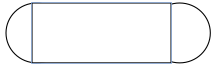96 ft

4π + 24

40 ft.

8π + 24

8π + 24

Explanation:

The shape of the garden consists of a rectangle and two semi-circles. Since they are building a fence we need to find the perimeter. The perimeter of the length of the rectangle is 24. The perimeter or circumference of the circle can be found using the equation C=2π(r), where r= the radius of the circle. Since we have two semi-circles we can find the circumference of one whole circle with a radius of 4, which would be 8π.

### Example Question #6 : How To Find Circumference

The diameter of a circle is defined by the two points (2,5) and (4,6). What is the circumference of this circle?

2.5π

π√2.5

π√5

π√5

Explanation:

We first must calculate the distance between these two points. Recall that the distance formula is:√((x2 - x1)2 + (y2 - y1)2)

For us, it is therefore: √((4 - 2)2 + (6 - 5)2) = √((2)2 + (1)2) = √(4 + 1) = √5

If d = √5, the circumference of our circle is πd, or π√5.

### Example Question #1 : How To Find Circumference

If a circle has an area of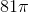, what is the circumference of the circle?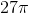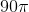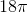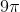Explanation:

The formula for  the area of a circle is πr2. For this particular circle, the area is 81π, so 81π = πr2. Divide both sides by π and we are left with r2=81. Take the square root of both sides to find r=9. The formula for the circumference of the circle is 2πr = 2π(9) = 18π. The correct answer is 18π.

### Example Question #8 : How To Find Circumference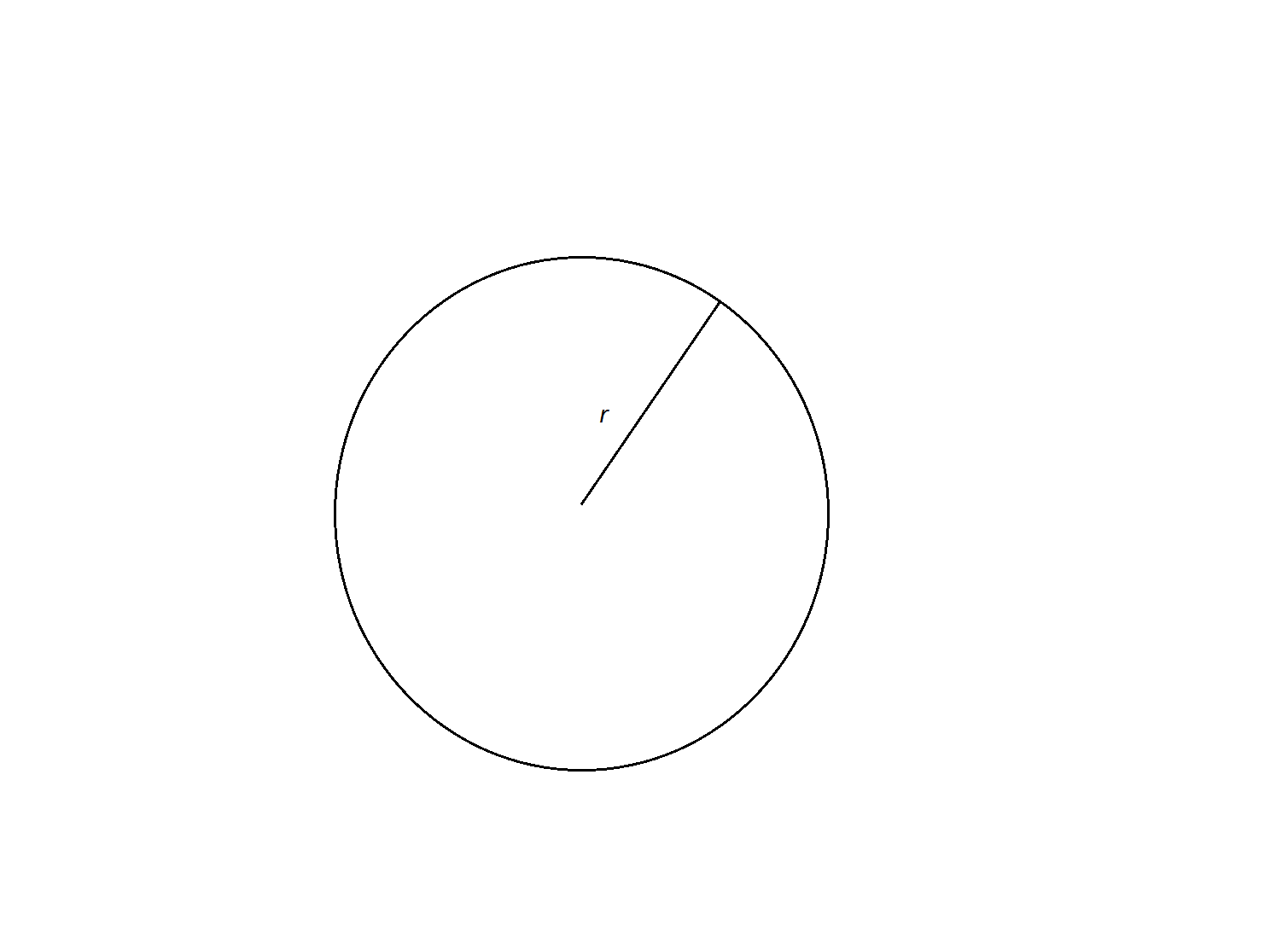Find the circumference of a circle with a radius of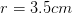.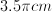Not enough information to solve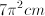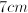Explanation:

In order to find the circumference, we will use the formula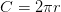.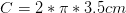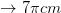### Example Question #9 : How To Find Circumference

This figure is a circle with a radius of 3 cm.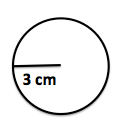What is the circumference of the circle (cm)?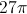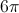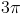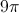Explanation:

In order to find the circumference of a circle (which is the perimeter or distance around the circle), you must double the radius and multiply by pi (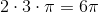).

### Example Question #10 : How To Find Circumference

What is the circumference of a circle with a radius of 4?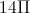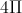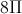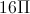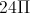The equation for the circumference of a circle is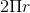, so by substituting the given radius into the equation, we get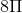.